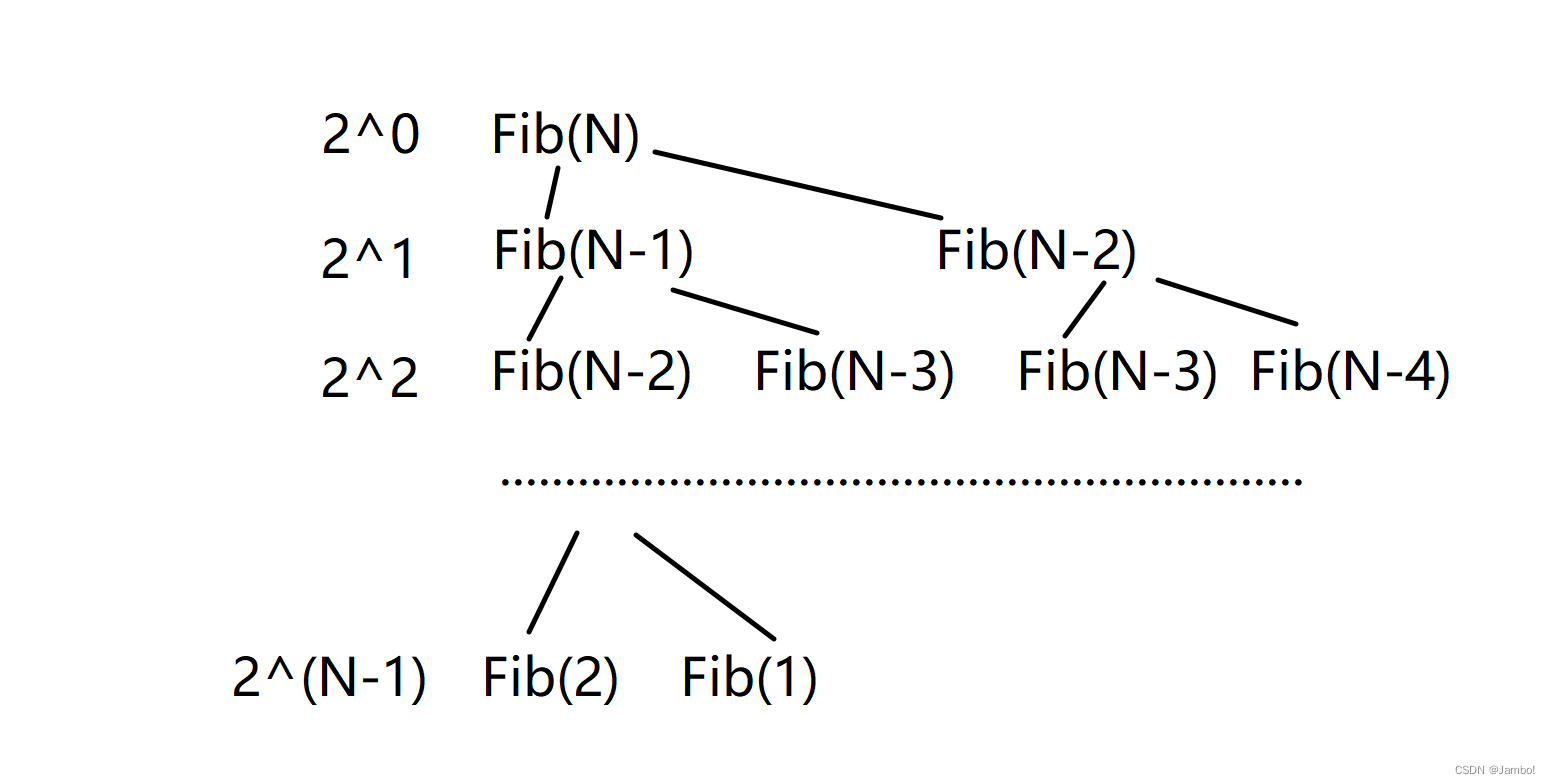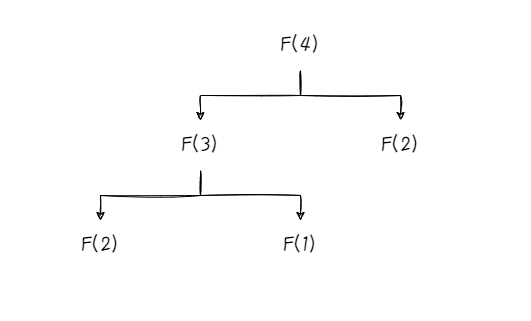+关注继续查看

# 递归写法

f(0) = 1

f(1) = 1

f(n) = f(n-1)+f(n-2)(n>3)

long long feibonahi(int n)
{
if (n <= 2)
{
return 1;
}
else
{
return feibonahi(n - 1) + feibonahi(n - 2);
}
}#include <stdio.h>
#include <time.h>
long long Fib(int n)
{
if (n <= 2)
{
return 1;
}
else
{
return Fib(n - 1) + Fib(n - 2);
}
}
int main()
{
int begin1 = clock();
Fib(10);
int end1 = clock();
int begin2 = clock();
Fib(20);
int end2 = clock();
int begin3 = clock();
Fib(30);
int end3 = clock();
int begin4 = clock();
Fib(40);
int end4 = clock();
int begin5 = clock();
Fib(50);
int end5 = clock();
printf("end1:%d\n", end1 - begin1);
printf("end2:%d\n", end2 - begin2);
printf("end3:%d\n", end2 - begin3);
printf("end4:%d\n", end4 - begin4);
printf("end5:%d\n", end5 - begin5);
return 0;
}N==10，2^N = 1024

N==20，2^N = 100万

N==30，2^N = 10亿

N==40，2^N = 1万亿

N==50，2^N = 很大很大的数

int feibonahi2(int n)
{
if (n == 1 || n == 2)
{
return 1;
}
int a = 1;
int b = 1;
int c = 1;
while (n > 2)
{
c = a + b;
a = b;
b = c;
n--;
}
return c;
}|
1月前

8 0
|
3月前
|

46 1|
5月前
|

42 0
|
10月前

48 0
|
10月前
|

68 0|
Java Python

49 0

77 0

94 0